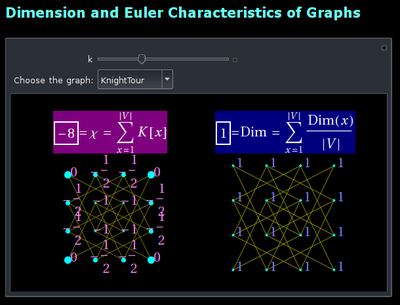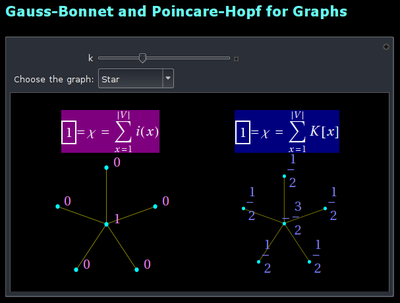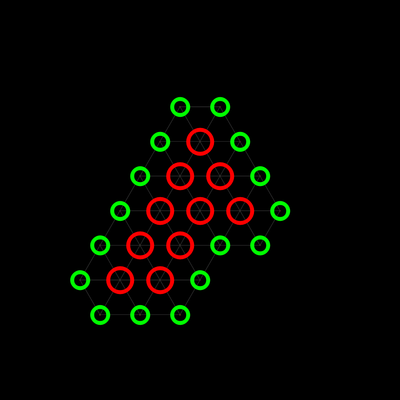# Code

Update November 2020:
< br /> A blog entry in the Wolfram community page. Mathematica Notebook .nb. In the last couple of years, I added the code directly to the papers so that it can be grabbed from the ArXiv, (where the LaTeX source code remains available). All of my programs are by default in the public domain (but of course, it should be obvious (as with the use of any other mathematical writing, idea or talk material), that the re-use (even in modified form) should be acknowledged).
>
 June 10: Some code to the ArXiv paper.This is code base used for many of the computations in papers 2 and 3. It allows to compute curvature, dimension and Euler characteristic for a general graph. It is available as a Wolfram Demonstration project: "Dimension and Euler Characteristics of graphs". I have here also a local version (CDF file January 8, 2012) Like any Demonstration projects the file is open source. You can download the source, try it out and look under the hood or modify.This contains also code base for the computations in paper 4. It contains code to compute the index at any point. The demonstration project illustrates with some small graphs how the sum of the indices is the Euler characteristic. Like Gauss-Bonnet for graphs, Poincare-Hopf for graphs is very general. Both curvature and index have simple definitions, much simpler than the continuum versions. Wolfram Demo version, local (CDF file January 9, 2012).hexregions.m ( July 2, 2009) This is code base which generated most of the pictures for the first paper. It was written in Mathematica 7 and was heavily based on Euclidean implementation. I did not bother to rewrite graph theoretically, but it still runs today with Mathematica 8.
Dimension and Euler Characteristics of Graphs from the Wolfram Demonstrations Project by Oliver Knill
Gauss-Bonnet and Poincaré-Hopf for Graphs from the Wolfram Demonstrations Project by Oliver Knill